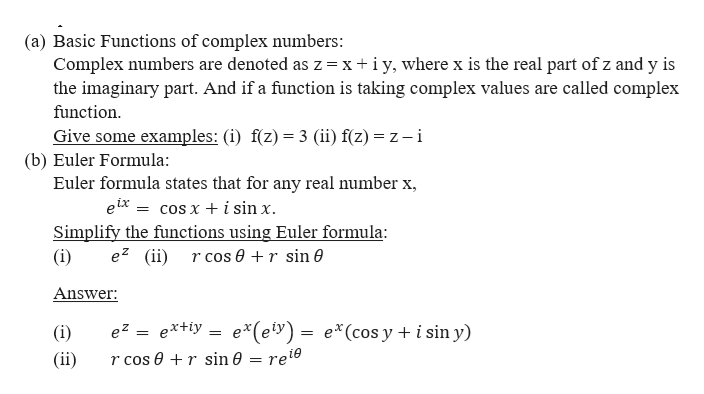# give 2 examples of questions and answers for each material namelya. basic functions of complex numbers,b. euler formulation,c. roots and rank of complex numbers, d. exponential and trigonometric functions of complex numbers,e. hyperbolic functions,f. logarithm.

Question

give 2 examples of questions and answers for each material namely

a. basic functions of complex numbers,

b. euler formulation,

c. roots and rank of complex numbers, d. exponential and trigonometric functions of complex numbers,

e. hyperbolic functions,

f. logarithm.

check_circleExpert Solution
Step 1

Hi there! You seem to have uploaded many sub-parts. As per our norm we will be answering only first 3 sub-parts. If you want to get the solutions for other sub-parts the kindly re-post the question by specifying them.

Step 2

We need to give 2 examples of questions and answers...help_outlineImage Transcriptionclose(a) Basic Functions of complex numbers: Complex numbers are denoted as z x iy, where x is the real part of z and y is the imaginary part. And if a function is taking complex values are called complex function Give some examples: (i) f(z) 3 (ii) f(z) z - i (b) Euler Formula Euler formula states that for any real number x, elxcos x + i sin x. Simplify the functions using Eul e2 (iir cos0 + r sin0 ormula (i) Answer e*(ey) e*(cos y i sin y) extiy (i) = = (ii) r cos er sin 0 reie fullscreen

### Want to see the full answer?

See Solution

#### Want to see this answer and more?

Solutions are written by subject experts who are available 24/7. Questions are typically answered within 1 hour*

See Solution
*Response times may vary by subject and question
Tagged in

### Math